Home > GC > Chapter 6 > Lesson 6.1.1 > Problem6-7

6-7.
1. For each of the triangles below, find x. Homework Help ✎

1.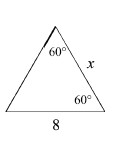2.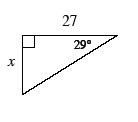3.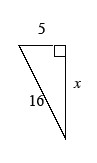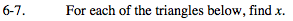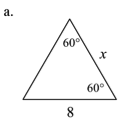Find the measure of the unknown angle.
What does this measure tell you about the lengths of the sides?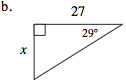Which trigonometric ratio can you use to find the unknown side?

$\text{tan 29^{\circ} =}\frac{x}{27}$

27 tan 29° = x

x ≈ 14.9663 feet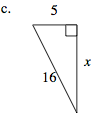Use the Pythagorean Theorem to find the length of the unknown side.